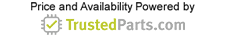MEMS and Sensors

# Design Notebook: Linearization of a Wheatstone Bridge

23 June 2015

Wheatstone bridge circuits have been in the field for a long time and are still among the first choices for front-end sensors. Whether a bridge is symmetrical or asymmetrical, balanced or unbalanced, it can be used to accurately measure an unknown impedance. In fact, the simplicity and effectiveness of a bridge circuit makes it useful for monitoring temperature, mass, pressure, humidity, light and other analog properties in industrial and medical applications.

The Wheatstone bridge has a single impedance-variable element that is inherently nonlinear away from the balance point. Bridge circuits are commonly used to detect the temperature of a boiler, chamber or a process situated perhaps hundreds of feet away from the actual circuit. Usually a sensor element--typically a resistance temperature detector (RTD), a thermistor or a thermocouple--is situated in the monitored environment to provide information about the change of resistance with change in temperature.

This article will consider the resistance-variable element in a Wheatstone bridge, examine its behavior and explain how to linearize the bridge circuit to optimize performance. Note that while the term “bridge” is generally used in this article, the focus is on circuit design for a Wheatstone bridge.

Single Variable-resistance Wheatstone Bridge

Variable-resistance Wheatstone bridge circuits perform most of the front-end tasks in a design. They use inexpensive, accurate discrete parts. By incorporating an RTD element, the bridge’s inherent resistance variations are kept within accepted linearity and tolerance limits, depending on the characteristics of the RTD.

RTD devices are provided with a detailed data sheet characterizing their behavior with look-up tables and even transfer function equations down to four or more orders of error-compensating terms. To ensure a high-precision system, designers must consider both the inherent nonlinearity of the RTD element and that of the Wheatstone bridge. Now it is necessary to calibrate the front-end and linearize the front-end at the microcontroller side. Increasing the order of the equation in the microcontroller is going to improve the linearity. A typical bridge circuit (Figure 1) detects milliohms of changes in resistance (DR).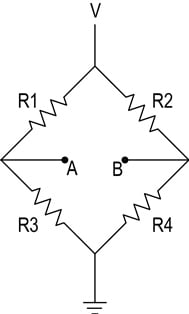Figure 1. A typical bridge with nodes A and B sensing output voltage from a change in resistance (DR).

Assuming that R1 = R2 = R3 = R4 = R in Figure 1, the bridge is balanced with nodes A and B at a constant V/2 (volts) with respect to ground and with a differential voltage of 0 V across nodes A and B (VAB). When there is a change in resistance (DR) in the R3 arm, then the output differential voltage created is:

When R1 = R2 = R3 = R4 = R, the bridge is balanced.

For a single variable-resistance element, for R3 = R + DR and R1 = R2 = R4 = R:

Equation 2 suggests that increasing the constant supply voltage, V, to the bridge will increase the output voltage, that is, the swing range across the bridge. This also suggests that having a dual supply across the four-legged resistance arrangement could be helpful not only to increase the range, but also to help maintain a 0 V common-mode voltage across the AB nodes.

The voltage VAB is usually amplified by a subsequent amplifying stage, typically a differential amplifier. There is a caveat, however. Changing the common-mode voltage across VAB adds more error and complexity in the amplifying second stage. This is usually realized as an instrumentation-quality differential amplifier. Therefore, a common-mode voltage centered on 0 V is preferable and easier to manage.

Figure 2 illustrates the natural tendency of the bridge’s single variable element: inherent nonlinearity in its transfer function.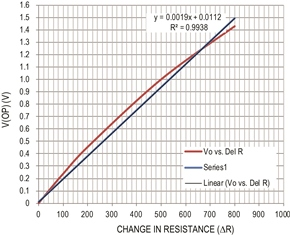Figure 2. V-AB vs. the change in DR, the effect of the bridges nonlinearity from 800W of resistance change. Trend line added for comparison.

Look closer at the trend line in the figure. The curve’s absolute deviation from an ideal straight line, or the linearity error, is about 0.62%. This is obtained by comparing the curved trend line with the line of best fit, that is, the straight line closest to the curve. In this way, we quantify the worst-case linearity error for the above curved line. In some cases 0.62 % is not acceptable. What follows is a way to achieve better than 0.1% accuracy.

Besides the inherent nonlinearity of the bridge, the designer must also manage the nonlinearity of the temperature sensor element, RTD or thermistor discussed earlier. When sensing the differential voltage across the nodes A and B, the instrumentation amplifier (Figure 3) has a common-mode voltage of V/2. The amplifier is usually a differential amplifier with four resistors or a three-op-amp instrumentation amplifier integrated in a single package.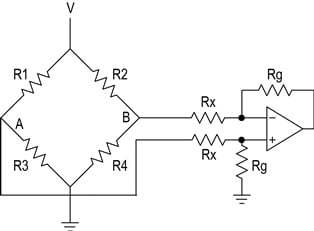Figure 3. An instrumentation amplifier connected to the original bridge circuit of Figure 1 .

When a differential amplifier is used, the nodes A and B are connected to the amplifier’s input gain-setting resistors, as shown in Figure 3. The choice of the op amp and input resistors is important as this path direwcts current away from the bridge, hence affecting the accuracy.

Also, the choice of resistors affects the bridge performance, as even 0.1% tolerance resistors used with the amplifier provide only 60 dB of common-mode rejection.

Linearize Bridge Output Without Instrumentation Amplifier

From the previous discussion, it seems logical to have dual supplies across the resistor bridge to increase the dynamic range, and to have the sensing nodes centered around the 0 V common-mode voltage. The advantage of this design is that the transfer function from node B is going to be linear with a change in resistance. The range of output swing from the bridge is doubled compared to the output from the circuit in Figure 1.

The circuit implementation in Figure 4 uses two op amps to replace the more complex instrumentation amplifier. Now the linearized bridge output avoids the unnecessary current paths created by the differential amplifier. This circuit eases the design process compared to the circuit in Figure 3. The only issue here is having positive and negative supplies to the amplifiers which are providing the doubling of swing range. The added benefit is improved common-mode rejection performance as the second amplifier operates comfortably around 0 V.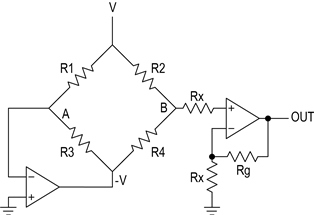Figure 4. This circuit replaces the complex instrumentation amplifier (Figure3) with two op amps.

From Figure 4, node A sees GND as it is the summing node of amplifier 1. Thus, a constant current of is forced through the R1|R3 branch, producing an equal and opposite voltage on the other side of the bridge with -V. When the single variable-resistance R3 changes (from R3 to R ±DR), then Ix (the change in current due to change in the resistance) flowing through this resistance produces a voltage V ±DV. A factor of this DV is manifested across node B by the balancing of the resistance bridge (for a balanced bridge, of course), as the current forced through resistor branch R2|R4 is equal to (V+ - (V- + DV))/(R3+R4). Since node B is centered at 0 V common mode, the voltage produced across node B is going to be applied to the noninverting input of the amplifier. Furthermore, filtering can be done on this gain stage to optimize the bandwidth and, therefore, make the noise level acceptable for the application.

At balance, when R1 = R2 = R3 = R4 = R, the voltage at nodes A and B is:When a single variable-resistive element (R3) changes by DR (R3 + DR):Since node A is at ground, the voltage at node B is: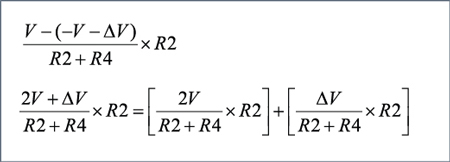And in a balanced bridge:The same equation can be written as:At the output of the noninverting op amp, the equation is:

Figure 5 shows the transfer function and its nonlinearity from the Figure 4 implementation.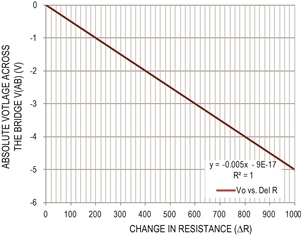Figure 5. Bridge output vs. change in resistance. Data are based on the design in Figure 4.

The absolute deviation from an ideal straight line (that is the linearity error) in Figure 5 is less than 0.02%. Improvement in absolute nonlinearity means that the full-scale error or the relative error is also going to improve.

There are no interacting resistive branches, so precision matching of resistors is not required. The variation of the Rx and Rg will only provide a gain error, which can be calibrated at the same time as the RTD device.

The above data suggest that this approach can be a viable implementation for 12-, 14-, 16- even 18-bit applications. The design is simple and little calibration is needed by the microcontroller. This circuit has, in fact, been widely used for many years.

To implement the Figure 4 circuit, a designer needs a dual-supply voltage for the front-end. This negative supply also needs additional board space and components, a requirement that often may not be a viable option if this is the only place in the entire system where the negative supply is needed. Low offset voltage, low-offset drift and low-noise performance are additional requirements for a high-precision bridge sensor.

Implementing the Bridge Design with a Dual Op Amp

What if the amplifier used in Figure 4 needs only one power supply and is capable of outputting bipolar voltages? Some single-supply amplifiers need headroom above ground, but a device that provides a true-zero output may be a fit for bridge sensors (Figure 6). A possibly ideal dual op-amp for this application integrates charge-pump circuitry that generates the negative voltage rail in conjunction with external capacitors. This allows the amplifier to operate from a single +4.5 V to +15 V power supply, but it is as effective as a normal dual-rail ±4.5 V to ±15 V amplifier.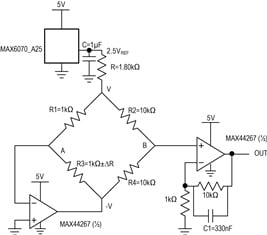Figure 6. The MAX44267 precision, low-noise, low-drift, dual op amp offers a true-zero output from a single supply.

One such amplifier is the MAX44267, which can be implemented in the circuit of Figure 6 with just one supply voltage (positive supply, VCC). The integrated negative VSS generator or charge pump generates a negative supply voltage. This architecture provides an advantage to the designer, because it eliminates the need for negative supply regulators and reduces board layout space and cost.

Figure 7 includes a voltage reference to generate a 2.5 VOUT reference. A dual op amp is used with the resistance bridge where R1 = R3 = 1 kW and R2 = R4 = 10 kW. An additional 1.8 kW is used in series to reduce the amount of current flowing through the bridge and to reduce power dissipation. The V(+) node becomes one-third the voltage reference’s output at a balanced condition. This is followed with second-stage amplification having a gain of 11 at the OUTA node.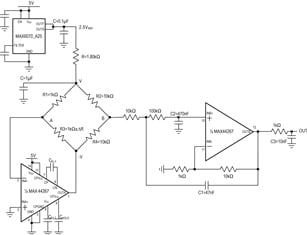Figure 7. The MAX44267 op amp operates from a single supply.

A Fluke RTD calibrator was used as the temperature-dependent resistance element (as PT1000) in place of R3; a temperature change from -50 C to +155 C is evaluated. For the given temperature change using a PT1000, the change in resistance(DR) is about 800 W and an equivalent range of 325 mV is effected (see Equation 4). Because amplifier 2 has an internal negative supply, it can accommodate this swing (-242mV to -83mV) at its input below ground, and it provides an output gain of 11.

Figure 7 utilizes a Sallen-Key filter in the second stage to filter the input signal to the required bandwidth (50Hz used in this case). Full-scale error accuracy within ±0.05% is obtained from the bridge output at node B without any calibration or trim. In this way the transfer function of the bridge circuit is made linear; improved performance of the front-end circuit is realized using MAX44267 in the subsequent section.

Test Measurements

1.Figure 8 shows the absolute bridge voltage output versus change in resistance (a linearity curve output), under 0.02%.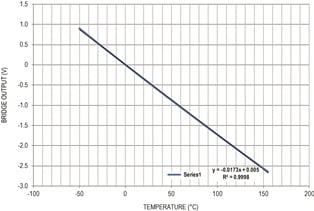Figure 8.The transfer curve shows the absolute voltage output vs. temperature for the Figure 7 circuit.

2. Figure 9 shows the gain error plot as percentage versus full-scale. The error curve shows small wriggles, in order of 0.002%, that are a combination of manual data plotting and measurement setup noise.Figure 9. Error % vs. full scale for the circuit in Figure 7.

3. Figure 10 shows the voltage noise density of the bridge plus amplifier: 115nV/ÖHz at 1kHz and 500nV/ÖHz under 50Hz. A 50 Hz filter was implemented in the second stage to remove the sensitivity to line noise.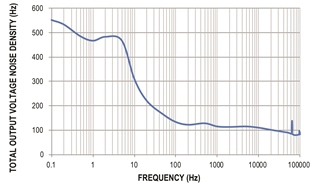Figure 10. Output noise density vs. frequency for the circuit in Figure 7.

4. Figure 11 shows the voltage noise(VP-P) of the bridge plus amplifier, 0.1Hz to 10Hz, 6mVP-P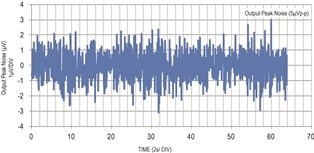Figure 11. Peak-to-peak voltage noise from 0.1Hz to 10Hz for the circuit in Figure 7.

References

1. Reference Data for Radio Engineers , Howard W. Sams & Co.inc (ITT)
2. Practical Design Techniques for Sensor Signal Conditioning, Section 2: Bridge Circuits by Walter Kester
3. Wheatstone Bridge Nonlinearity, Measurements Group Tech Note TN-507, 12/18/2000, http://www.thermofisher.com.au/Uploads/file/Environmental-Industrial/Process-Monitoring-Industrial-Instruments/Sound-Vibration-Stress-Monitoring/Stress-Analysis/VishayMM/technology/technotes/TN-507-Wheatstone-Bridge-Nonlinearity.pdf.

Ashwin Badri Narayanan is a Member of Technical Staff at Maxim Integrated. Stuart Smith joined Maxim Integrated in 2011 as a Product Definer. He has worked for more than 30 years as an analog and mixed-signal IC design engineer and has received eight patents during that time. Stuart has a BSC EE from Abertay University and is a Chartered Engineer.